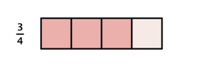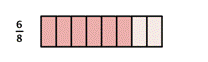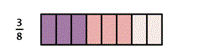## Multiplying Fractions

### Learning Outcomes

• Multiply two or more fractions
• Multiply a fraction by a whole number

## Multiply Fractions

Just as you add, subtract, multiply, and divide when working with whole numbers, you also use these operations when working with fractions.   There are many times when it is necessary to multiply fractions. A model may help you understand multiplication of fractions.

When you multiply a fraction by a fraction, you are finding a “fraction of a fraction.” Suppose you have $\dfrac{3}{4}$ of a candy bar and you want to find $\dfrac{1}{2}$ of the $\dfrac{3}{4}$:By dividing each fourth in half, you can divide the candy bar into eighths.Then, choose half of those to get $\dfrac{3}{8}$.In both of the above cases, to find the answer, you can multiply the numerators together and the denominators together.

### Multiplying Two Fractions

$\dfrac{a}{b}\cdot\dfrac{c}{d}=\dfrac{a\cdot c}{b\cdot d}=\dfrac{\text{product of the numerators}}{\text{product of the denominators}}$

### Multiplying More Than Two Fractions

$\dfrac{a}{b}\cdot\dfrac{c}{d}\cdot\dfrac{e}{f}=\dfrac{a\cdot c\cdot e}{b\cdot d\cdot f}$

### Example

Multiply $\dfrac{2}{3}\cdot\dfrac{4}{5}$

To review: if a fraction has common factors in the numerator and denominator, we can reduce the fraction to its simplified form by removing the common factors.

For example,

• Given $\dfrac{8}{15}$, the factors of $8$ are: $1, 2, 4, 8$ and the factors of $15$ are: $1, 3, 5, 15$.  $\dfrac{8}{15}$ is simplified because there are no common factors of $8$ and $15$.
• Given $\dfrac{10}{15}$, the factors of $10$ are: $1, 2, 5, 10$ and the factors of $15$ are: $1, 3, 5, 15$. $\dfrac{10}{15}$ is not simplified because $5$ is a common factor of $10$ and $15$.

Note that you can simplify the fractions first, before you multiplying them, to make your work easier. This allows you to work with smaller numbers when you multiply.

In the following video you will see an example of how to multiply two fractions, then simplify the answer.

Multiply $\dfrac{2}{3}\cdot\dfrac{1}{4}\cdot\dfrac{3}{5}$. Simplify the answer.